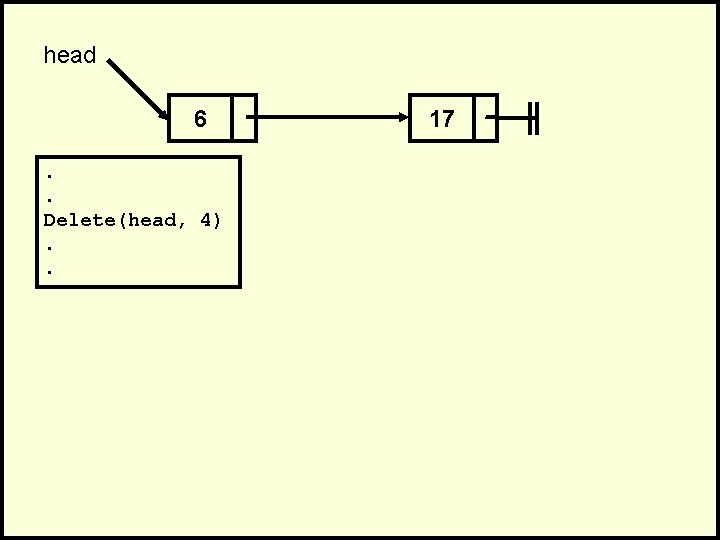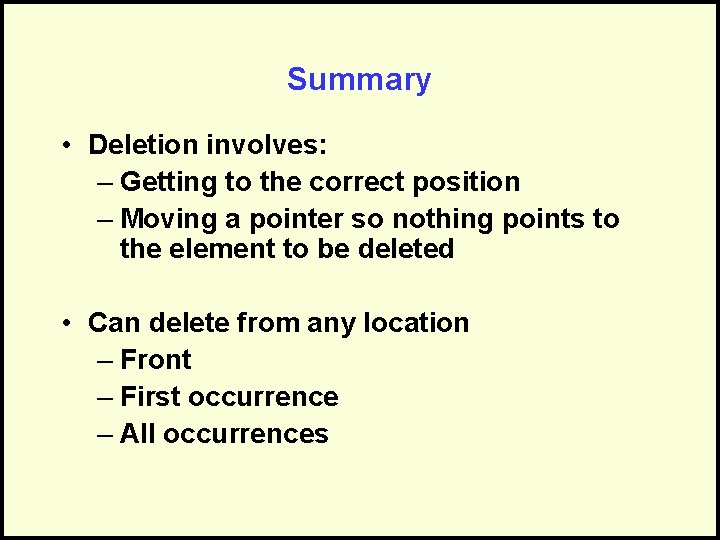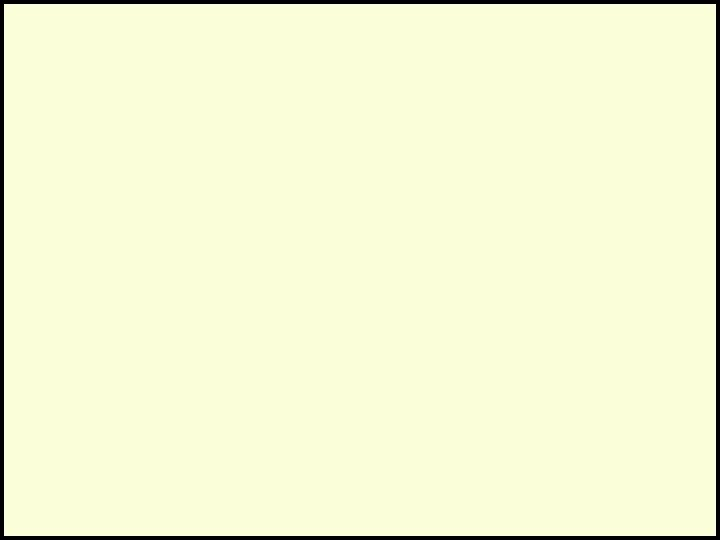• Slides: 89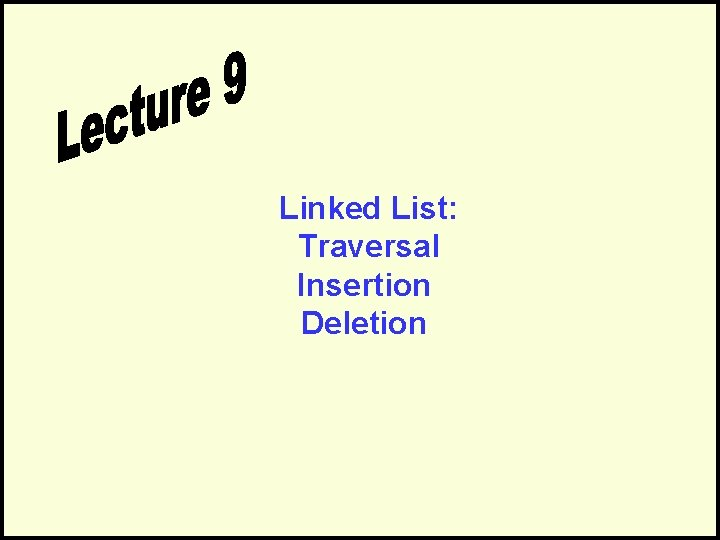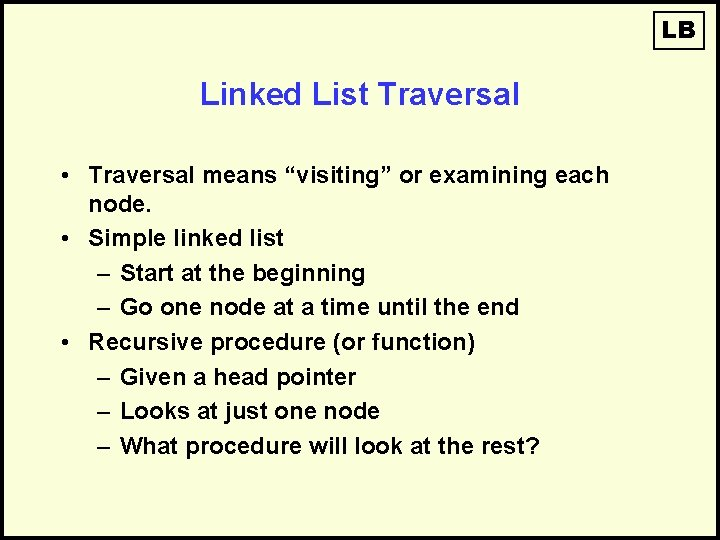LB Linked List Traversal • Traversal means “visiting” or examining each node. • Simple linked list – Start at the beginning – Go one node at a time until the end • Recursive procedure (or function) – Given a head pointer – Looks at just one node – What procedure will look at the rest?LB The Node Code Node definesa record data isoftype Num next isoftype Ptr toa Node endrecord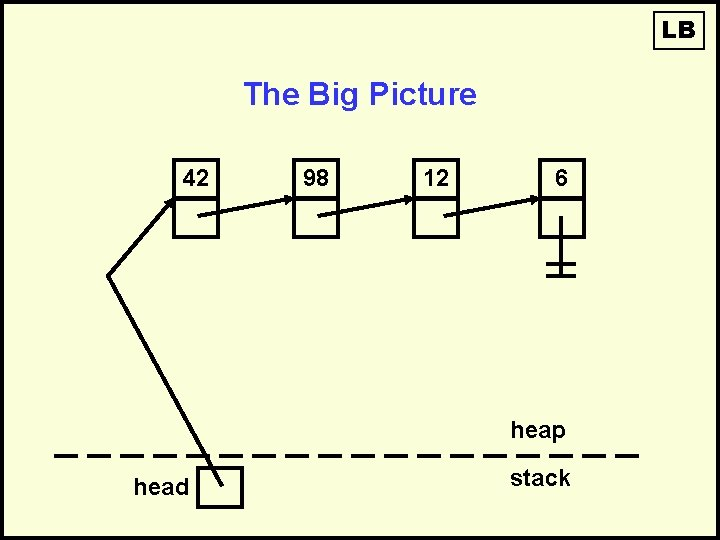LB The Big Picture 42 98 12 6 heap head stackLB The Little Picture 12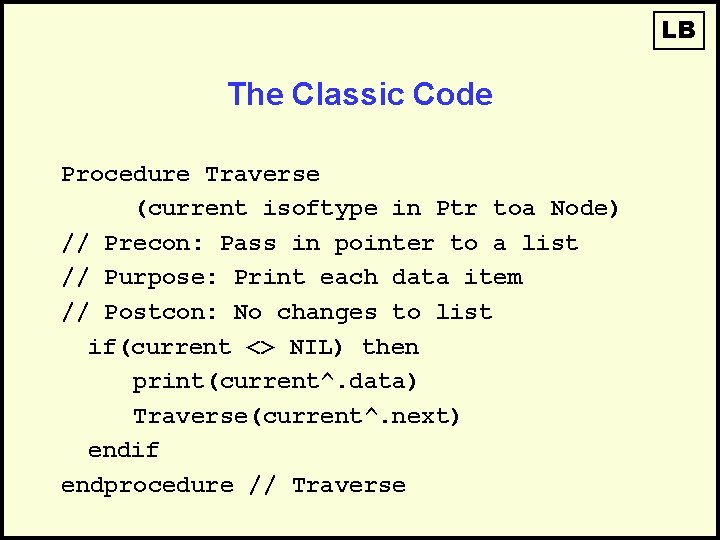LB The Classic Code Procedure Traverse (current isoftype in Ptr toa Node) // Precon: Pass in pointer to a list // Purpose: Print each data item // Postcon: No changes to list if(current <> NIL) then print(current^. data) Traverse(current^. next) endif endprocedure // TraverseLB The Big Picture 42 98 12 6 heap head stack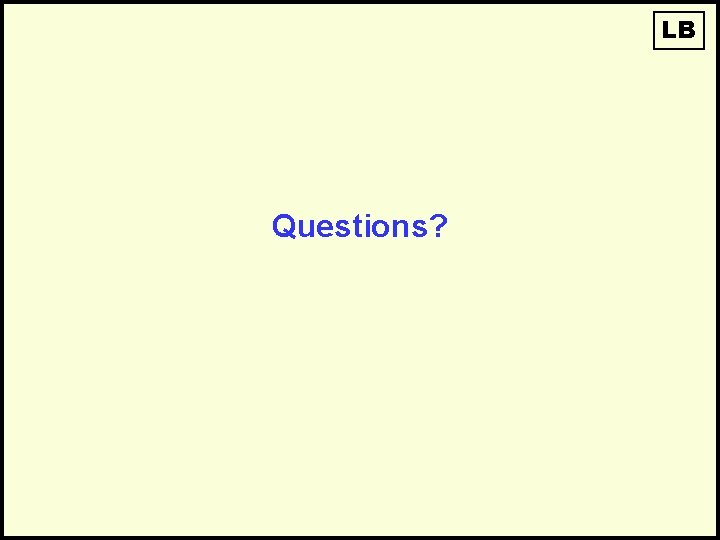LB Questions?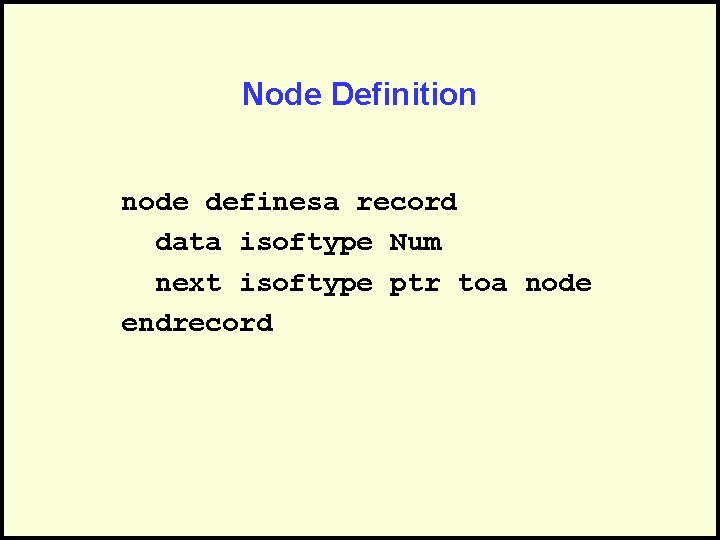Node Definition node definesa record data isoftype Num next isoftype ptr toa node endrecordThe Scenario • You have a linked list – Perhaps empty, perhaps not – Perhaps ordered, perhaps not head 48 17 142 • You want to add an element into the linked list //Adding an Element to a Linked List Involves two steps: • Finding the correct location • Doing the work to add the nodeFinding the Correct Location • Three possible positions: – The front – The end – Somewhere in the middle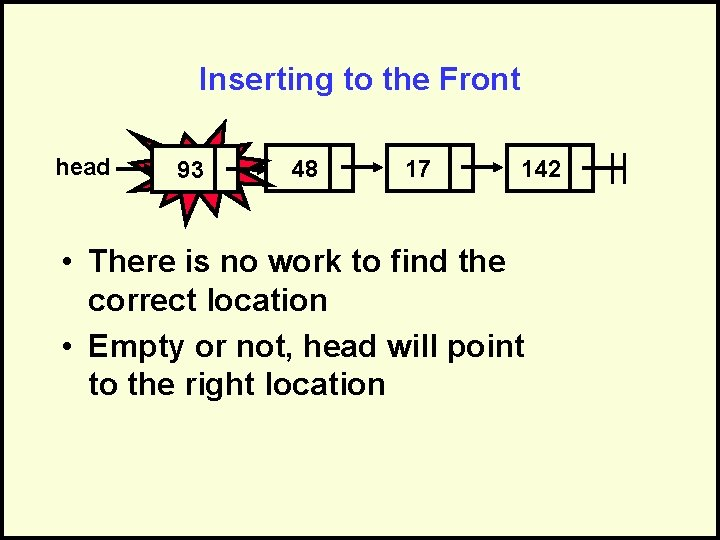Inserting to the Front head 93 head 48 17 142 • There is no work to find the correct location • Empty or not, head will point to the right location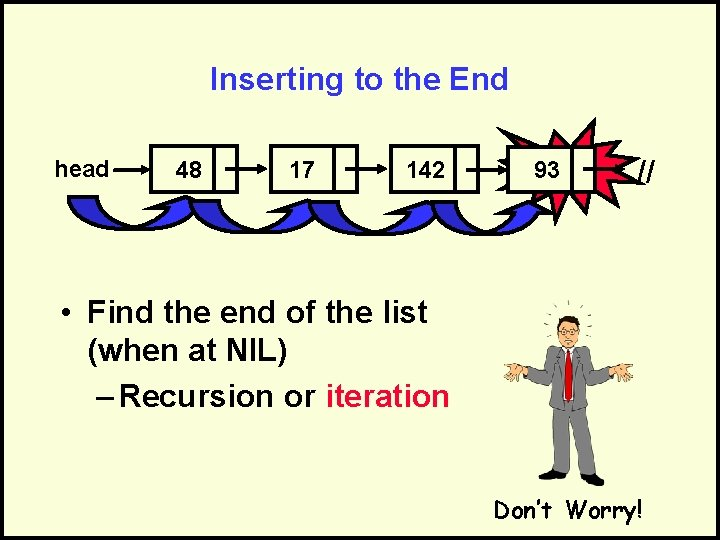Inserting to the End head 48 17 142 //93 // • Find the end of the list (when at NIL) – Recursion or iteration Don’t Worry!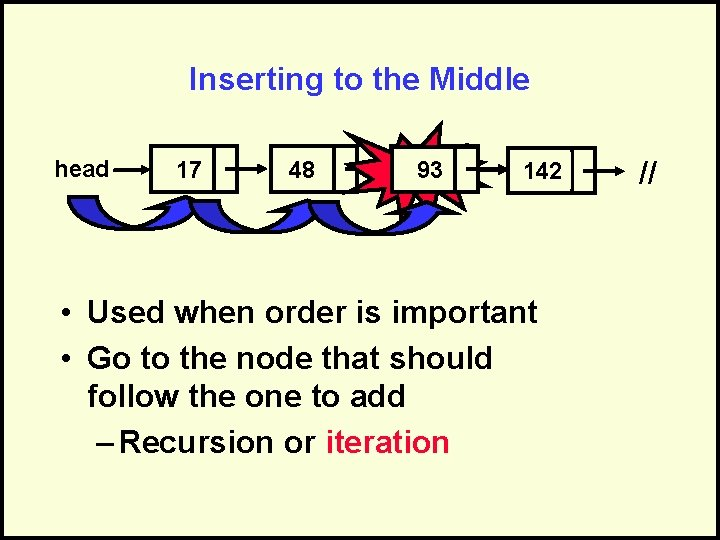Inserting to the Middle head 17 48 142 93 //142 • Used when order is important • Go to the node that should follow the one to add – Recursion or iteration //The Work to Add the Node • Create the new node • Fill in the data field • Deal with the next field – Point to nil (if inserting to end) – Point to current (front or middle) temp <- new(Node) temp^. data <- new_data temp^. next <- current <- temp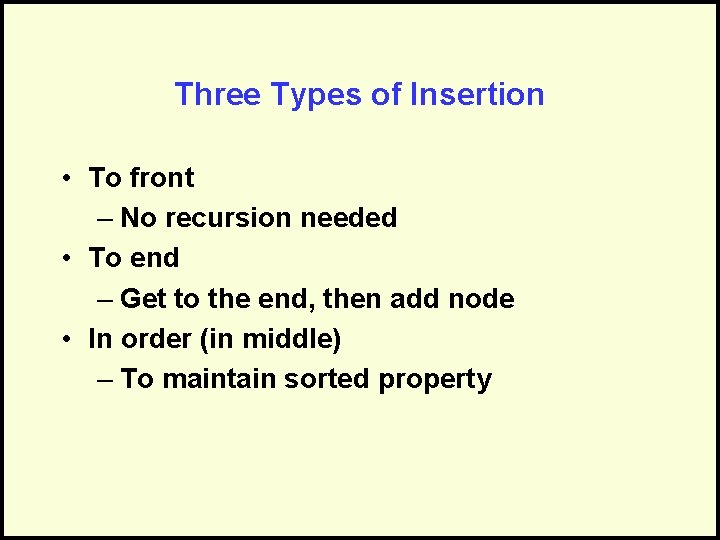Three Types of Insertion • To front – No recursion needed • To end – Get to the end, then add node • In order (in middle) – To maintain sorted propertyInserting at the Front of a Linked ListInserting to the Front of a Linked List • Need an in/out pointer parameter • Create new node • Fill in data • Make new node’s next pointer point to current • Update current to point to new nodehead Animated Insert to Front of Linked List 4 2 Current R new_data 42 17 2 temp procedure Insert (current iot in/out ptr toa Node, new_data isoftype in Num) temp isoftype ptr toa Node temp <- new(Node) temp^. data <- new_data temp^. next <- current <- temp endprocedure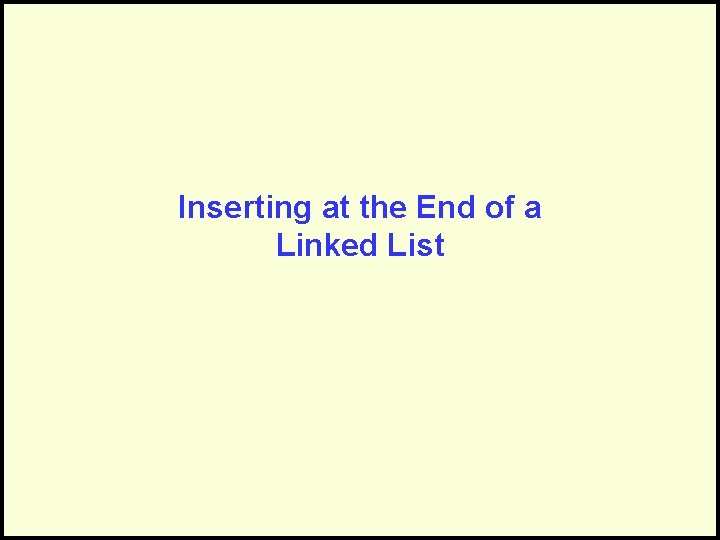Inserting at the End of a Linked ListInserting to End of a Linked List • Recursively traverse the list until at end • Then: – Create new node at current – Fill in data – Terminate the new node’s next pointer to point to NIL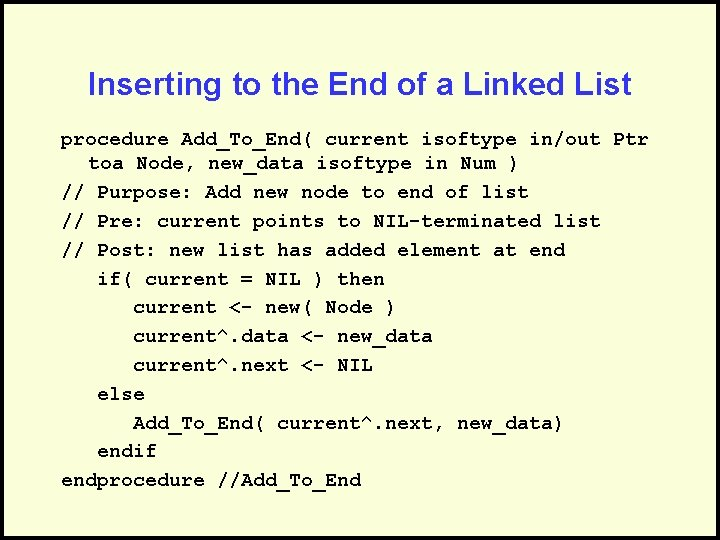Inserting to the End of a Linked List procedure Add_To_End( current isoftype in/out Ptr toa Node, new_data isoftype in Num ) // Purpose: Add new node to end of list // Pre: current points to NIL-terminated list // Post: new list has added element at end if( current = NIL ) then current <- new( Node ) current^. data <- new_data current^. next <- NIL else Add_To_End( current^. next, new_data) endif endprocedure //Add_To_End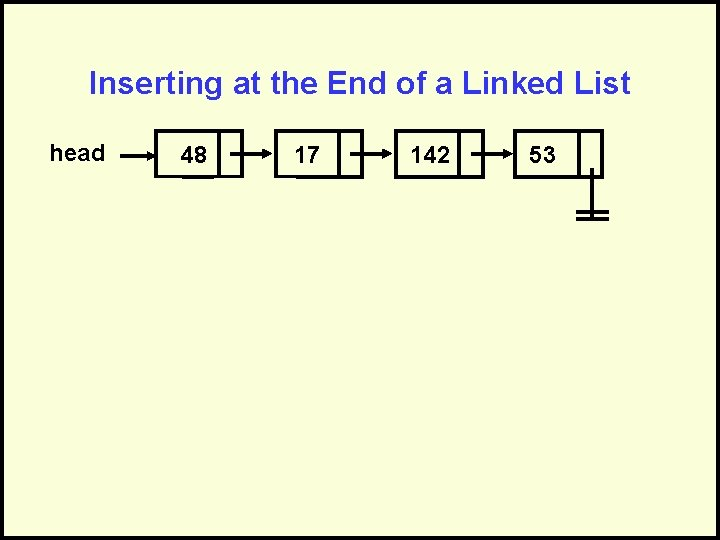Inserting at the End of a Linked List head 48 17 142 53 current R new_data 53Inserting in Order into a Linked List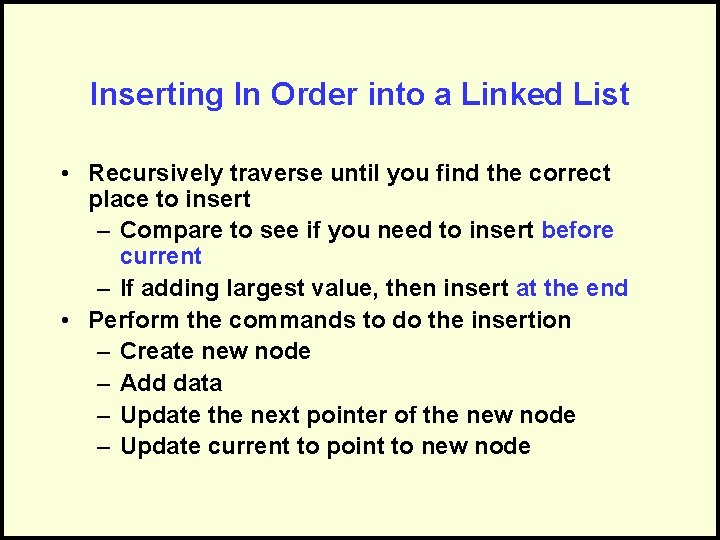Inserting In Order into a Linked List • Recursively traverse until you find the correct place to insert – Compare to see if you need to insert before current – If adding largest value, then insert at the end • Perform the commands to do the insertion – Create new node – Add data – Update the next pointer of the new node – Update current to point to new node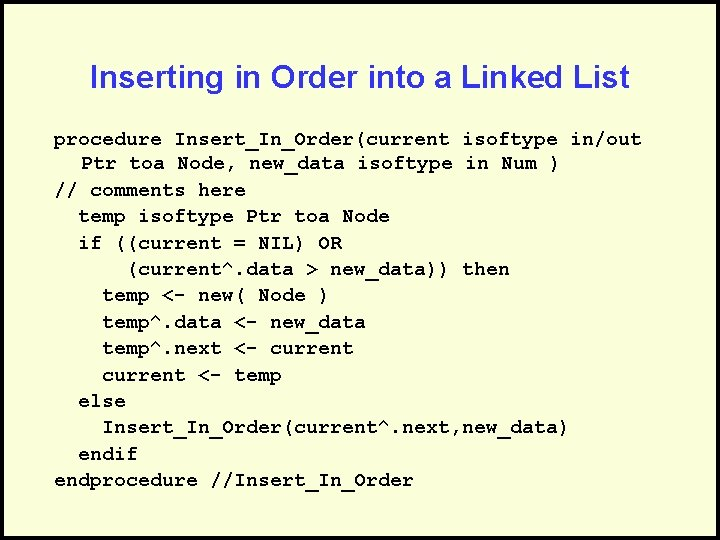Inserting in Order into a Linked List procedure Insert_In_Order(current isoftype in/out Ptr toa Node, new_data isoftype in Num ) // comments here temp isoftype Ptr toa Node if ((current = NIL) OR (current^. data > new_data)) then temp <- new( Node ) temp^. data <- new_data temp^. next <- current <- temp else Insert_In_Order(current^. next, new_data) endif endprocedure //Insert_In_Order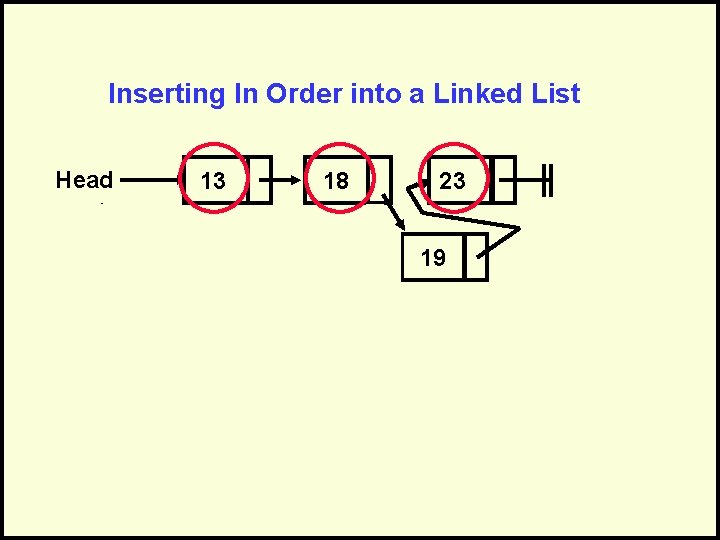Inserting In Order into a Linked List Head 13 18 23 19 current R R R new_data 19 temp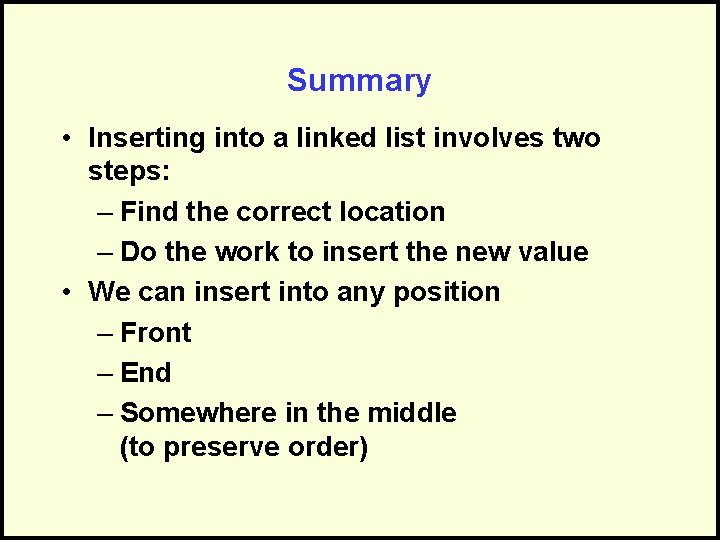Summary • Inserting into a linked list involves two steps: – Find the correct location – Do the work to insert the new value • We can insert into any position – Front – End – Somewhere in the middle (to preserve order)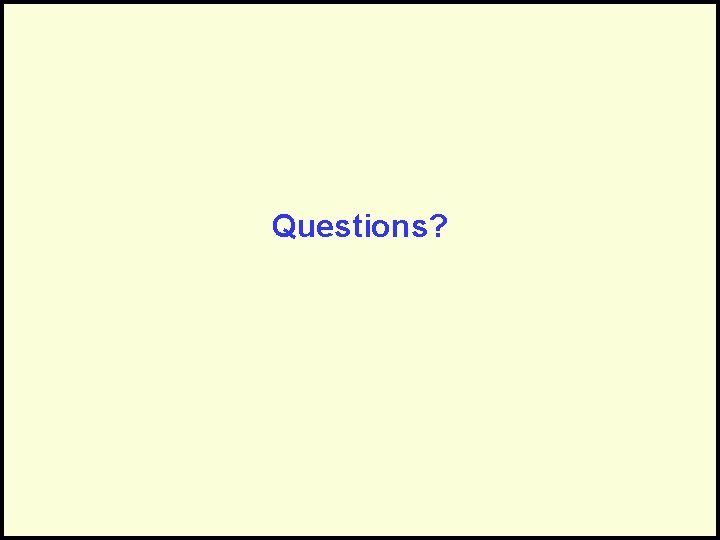Questions?Deleting an Element from a Linked List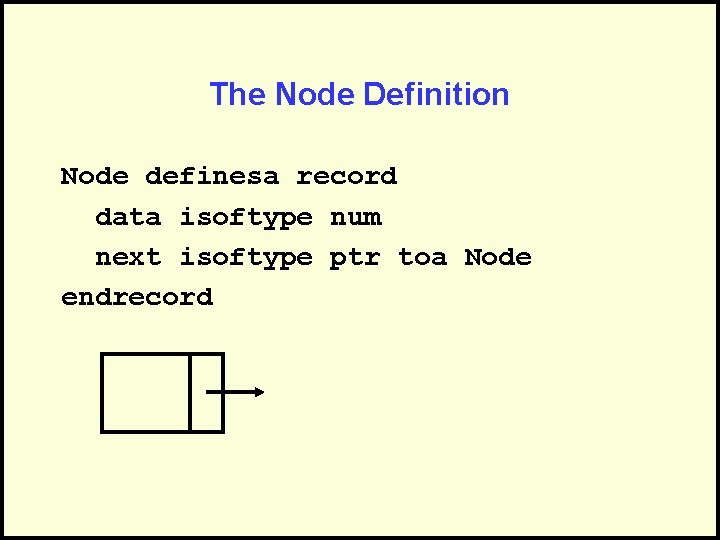The Node Definition Node definesa record data isoftype num next isoftype ptr toa Node endrecord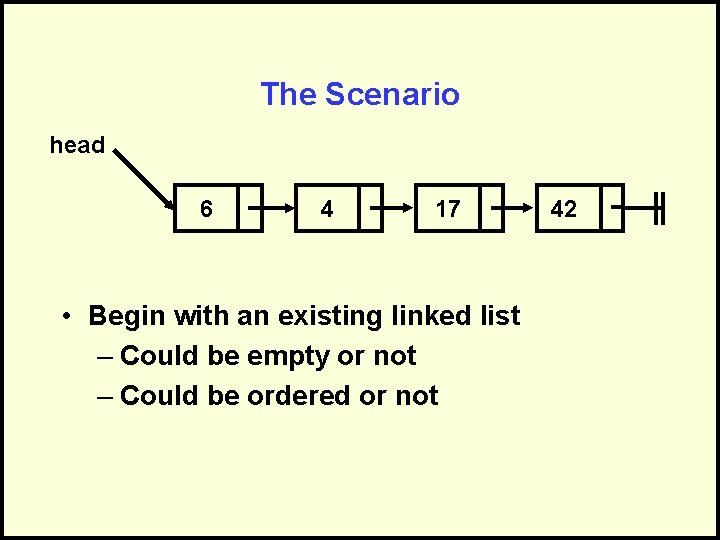The Scenario head 6 4 17 • Begin with an existing linked list – Could be empty or not – Could be ordered or not 42The Scenario head 6 4 17 • Begin with an existing linked list – Could be empty or not – Could be ordered or not 42The Scenario head 4 17 42 • Begin with an existing linked list – Could be empty or not – Could be ordered or not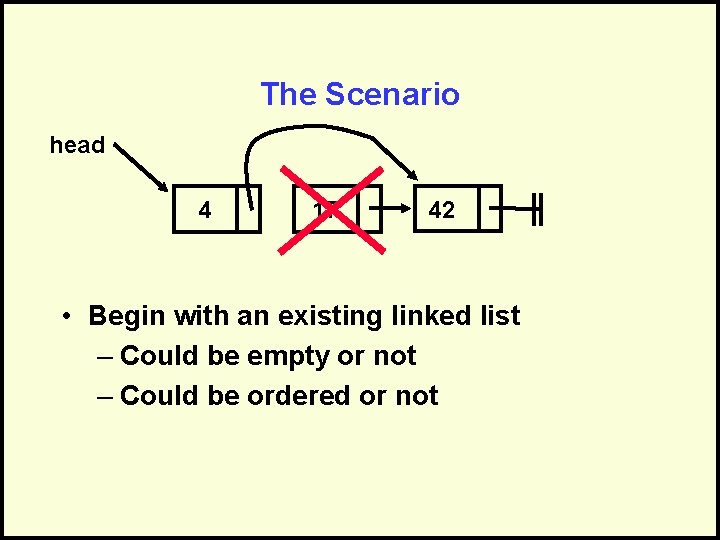The Scenario head 4 17 42 • Begin with an existing linked list – Could be empty or not – Could be ordered or not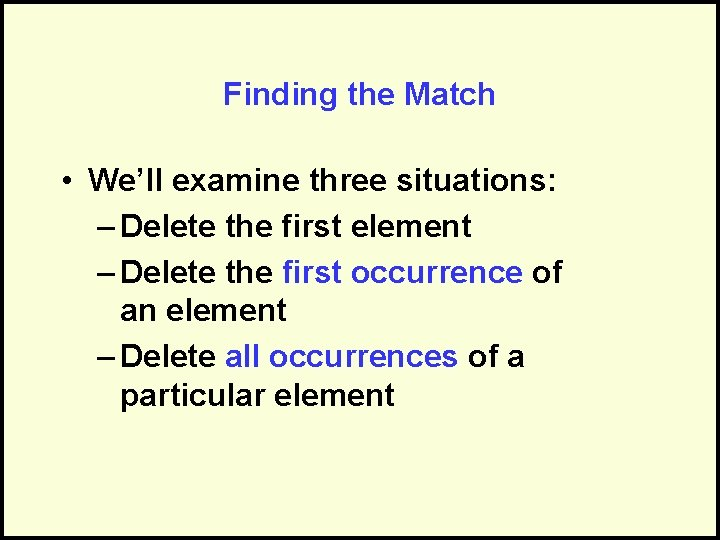Finding the Match • We’ll examine three situations: – Delete the first element – Delete the first occurrence of an element – Delete all occurrences of a particular elementDeleting the First Element • This can be done without any traversal/searching • Requires an in/out pointer procedure Delete. Front (current iot in/out ptr toa Node) // deletes the first node in the list if (current <> nil) then current <- current^. next endif endprocedure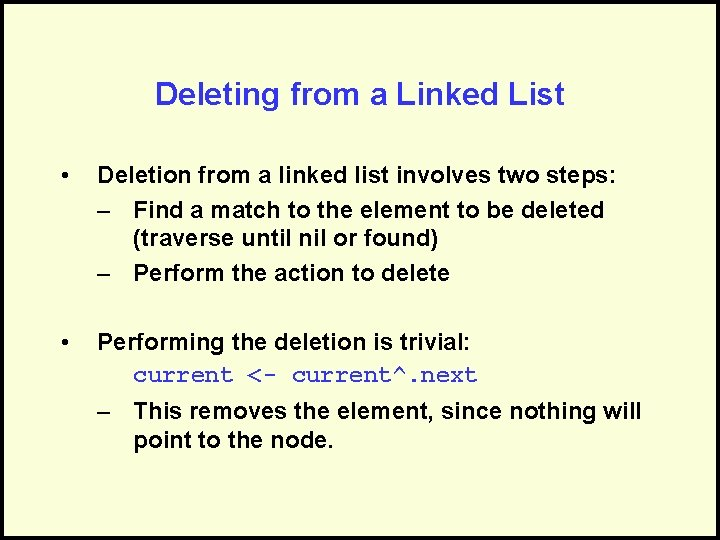Deleting from a Linked List • Deletion from a linked list involves two steps: – Find a match to the element to be deleted (traverse until nil or found) – Perform the action to delete • Performing the deletion is trivial: current <- current^. next – This removes the element, since nothing will point to the node.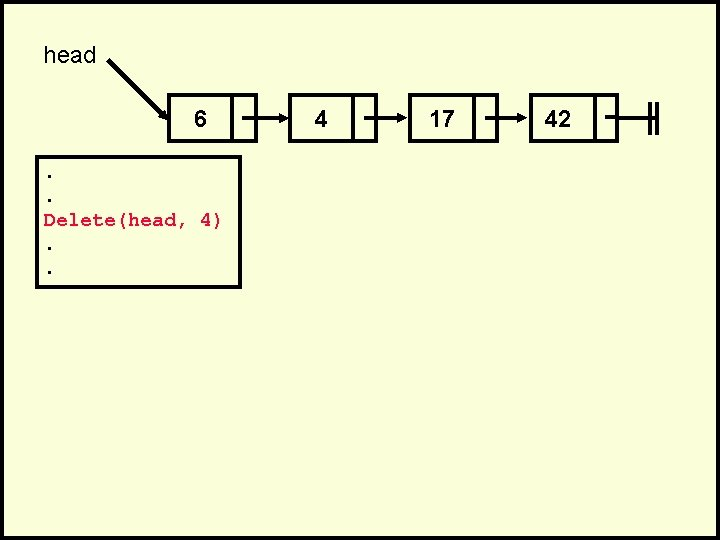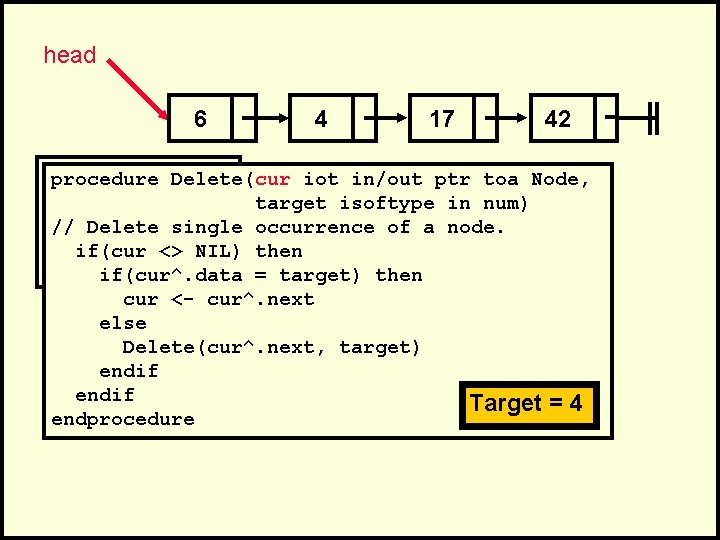head 6 4 17 42 . procedure Delete(cur iot in/out ptr toa Node, . target isoftype in num) Delete(head, 4) // Delete single occurrence of a node. . if(cur <> NIL) then. if(cur^. data = target) then cur <- cur^. next else Delete(cur^. next, target) endif endprocedure Target = 4head 6 4 17 42 . procedure Delete(cur iot in/out ptr toa Node, . target isoftype in num) Delete(head, 4) // Delete single occurrence of a node. . if(cur <> NIL) then. if(cur^. data = target) then cur <- cur^. next else Delete(cur^. next, target) endif endprocedure Target = 4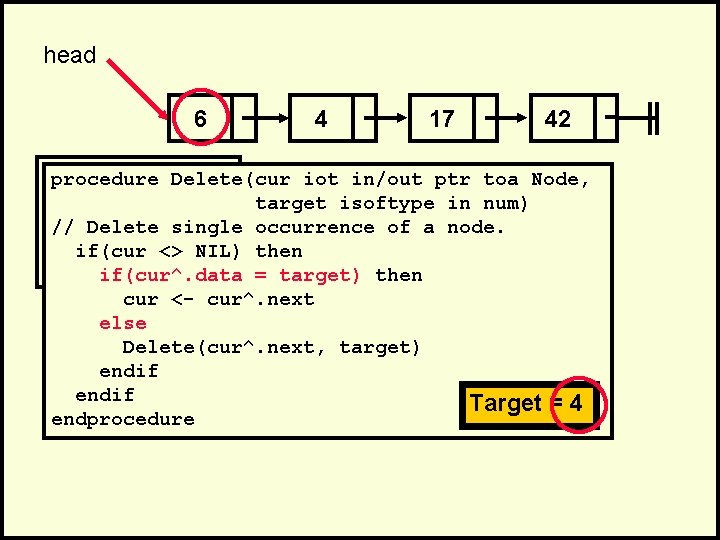head 6 4 17 42 . procedure Delete(cur iot in/out ptr toa Node, . target isoftype in num) Delete(head, 4) // Delete single occurrence of a node. . if(cur <> NIL) then. if(cur^. data = target) then cur <- cur^. next else Delete(cur^. next, target) endif endprocedure Target = 4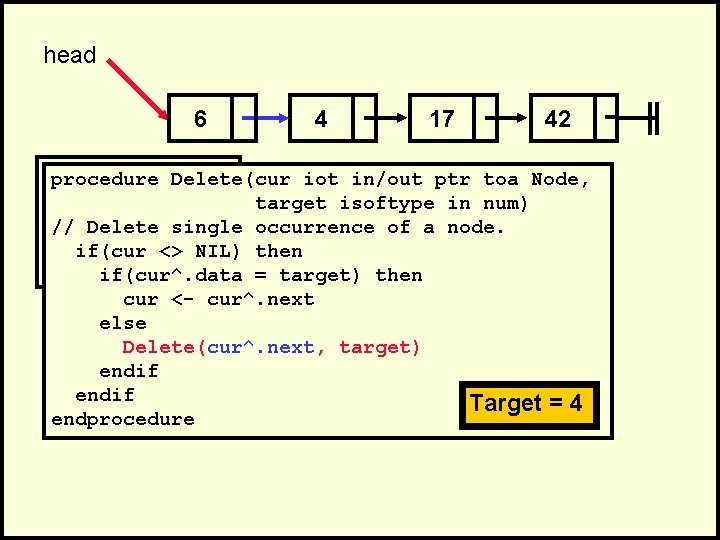head 6 4 17 42 . procedure Delete(cur iot in/out ptr toa Node, . target isoftype in num) Delete(head, 4) // Delete single occurrence of a node. . if(cur <> NIL) then. if(cur^. data = target) then cur <- cur^. next else Delete(cur^. next, target) endif endprocedure Target = 4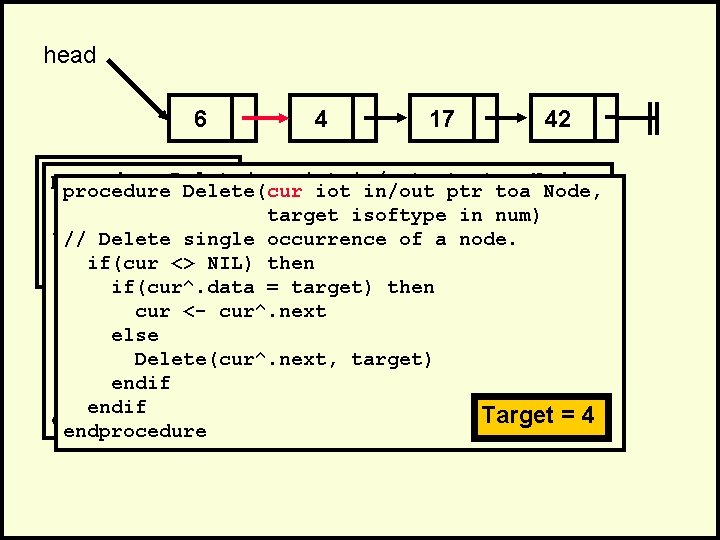head 6 4 17 42 . procedure Delete(cur iot in/out ptr toa Node, . procedure Delete(cur target isoftype in num) Delete(head, 4) // Delete single occurrence of a node. . // if(cur <> NIL) then. if(cur^. data = target) then cur <- cur^. next else Delete(cur^. next, target) endif Target ==44 endif Target endprocedurehead 6 4 17 42 . procedure Delete(cur iot in/out ptr toa Node, . procedure Delete(cur target isoftype in num) Delete(head, 4) // Delete single occurrence of a node. . // if(cur <> NIL) then. if(cur^. data = target) then cur <- cur^. next else Delete(cur^. next, target) endif Target ==44 endif Target endprocedure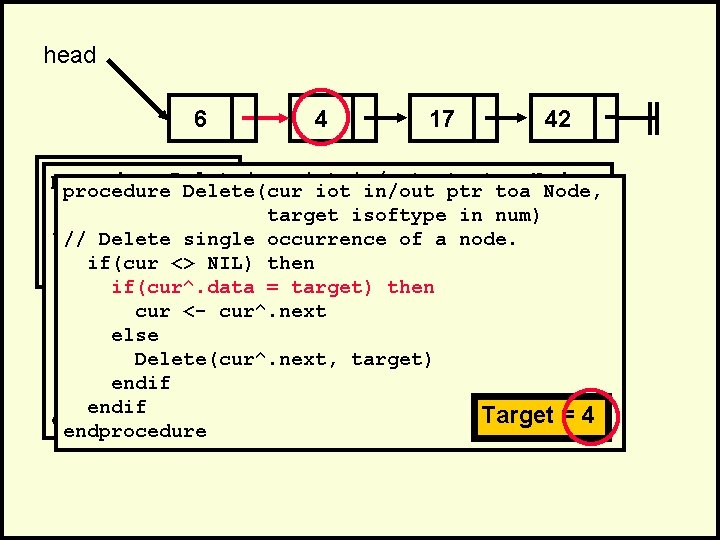head 6 4 17 42 . procedure Delete(cur iot in/out ptr toa Node, . procedure Delete(cur target isoftype in num) Delete(head, 4) // Delete single occurrence of a node. . // if(cur <> NIL) then. if(cur^. data = target) then cur <- cur^. next else Delete(cur^. next, target) endif Target ==44 endif Target endprocedure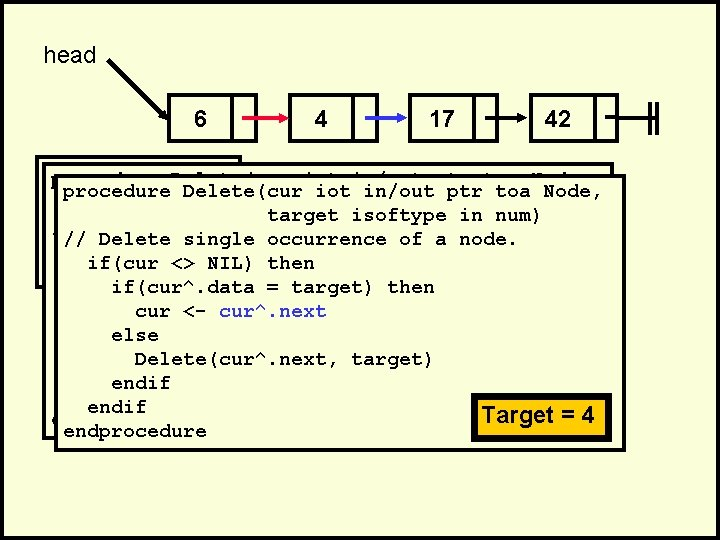head 6 4 17 42 . procedure Delete(cur iot in/out ptr toa Node, . procedure Delete(cur target isoftype in num) Delete(head, 4) // Delete single occurrence of a node. . // if(cur <> NIL) then. if(cur^. data = target) then cur <- cur^. next else Delete(cur^. next, target) endif Target ==44 endif Target endprocedure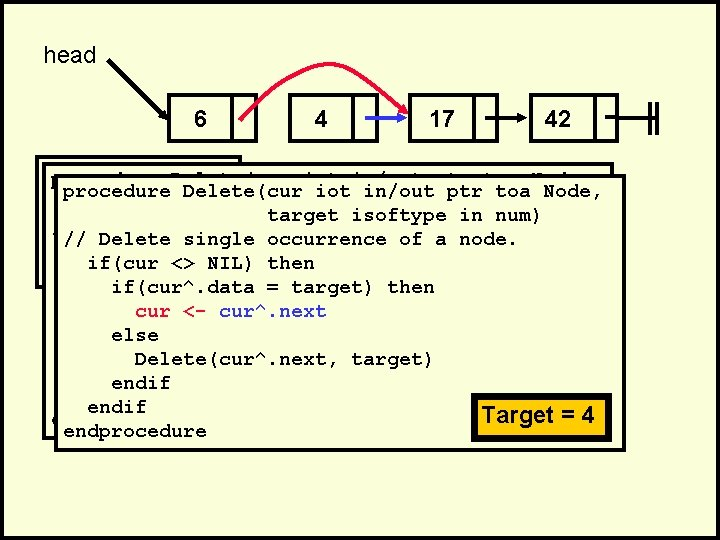head 6 4 17 42 . procedure Delete(cur iot in/out ptr toa Node, . procedure Delete(cur target isoftype in num) Delete(head, 4) // Delete single occurrence of a node. . // if(cur <> NIL) then. if(cur^. data = target) then cur <- cur^. next else Delete(cur^. next, target) endif Target ==44 endif Target endprocedurehead 6 4 17 42 . procedure Delete(cur iot in/out ptr toa Node, . procedure Delete(cur target isoftype in num) Delete(head, 4) // Delete single occurrence of a node. . // if(cur <> NIL) then. if(cur^. data = target) then cur <- cur^. next else Delete(cur^. next, target) endif Target ==44 endif Target endprocedurehead 6 17 42 . procedure Delete(cur iot in/out ptr toa Node, . procedure Delete(cur target isoftype in num) Delete(head, 4) // Delete single occurrence of a node. . // if(cur <> NIL) then. if(cur^. data = target) then cur <- cur^. next else Delete(cur^. next, target) endif Target ==44 endif Target endprocedure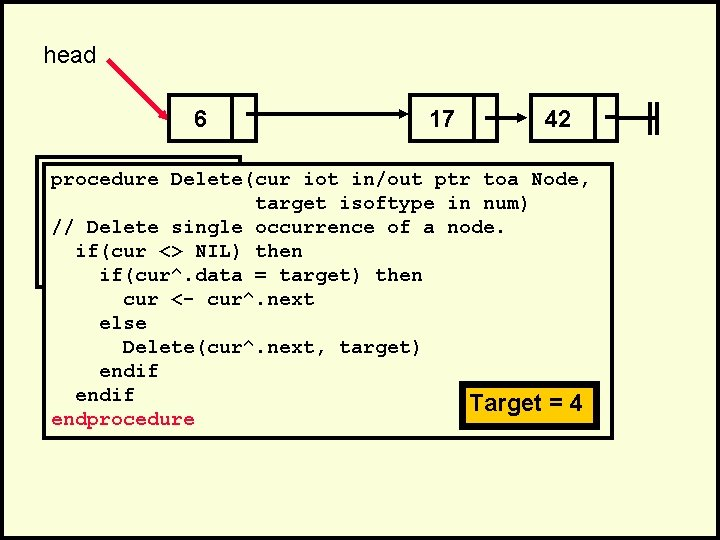head 6 17 42 . procedure Delete(cur iot in/out ptr toa Node, . target isoftype in num) Delete(head, 4) // Delete single occurrence of a node. . if(cur <> NIL) then. if(cur^. data = target) then cur <- cur^. next else Delete(cur^. next, target) endif endprocedure Target = 4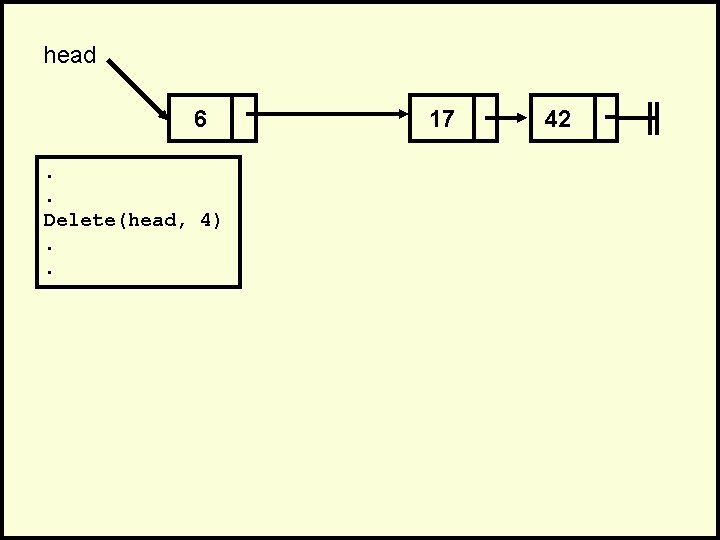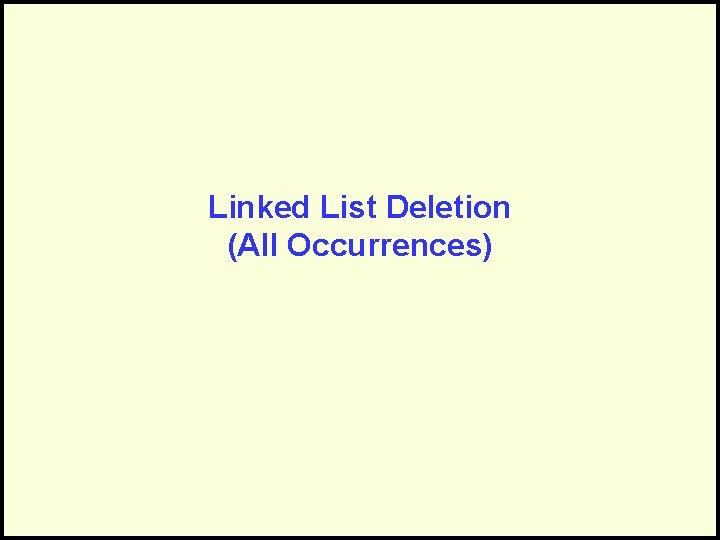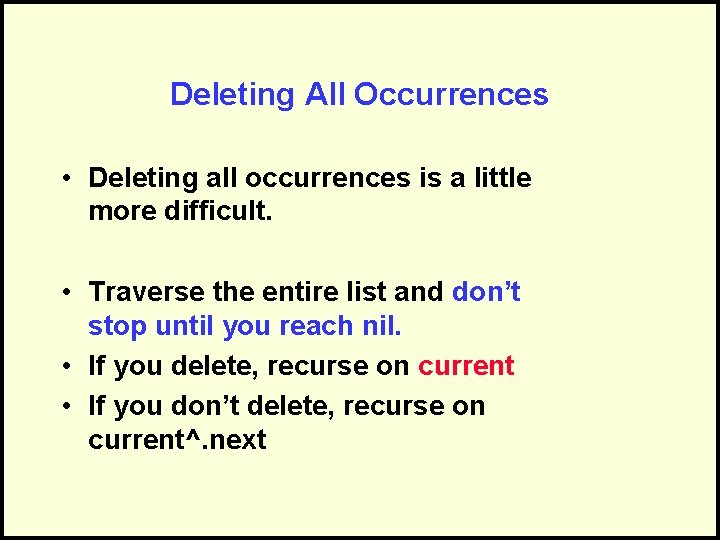Deleting All Occurrences • Deleting all occurrences is a little more difficult. • Traverse the entire list and don’t stop until you reach nil. • If you delete, recurse on current • If you don’t delete, recurse on current^. next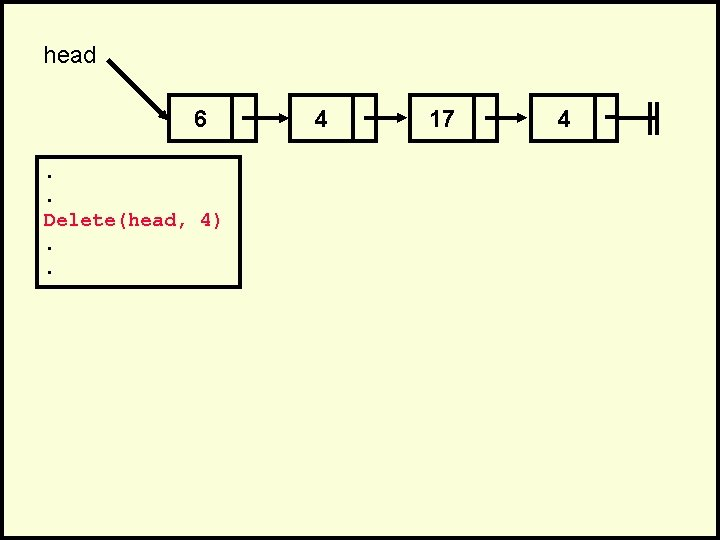head 6 4 17 4 . procedure Delete(cur iot in/out ptr toa Node, . target isoftype in num) Delete(head, // Delete all 4)occurrences of a node. . if(cur <> NIL) then. if(cur^. data = target) then cur <- cur^. next Delete(cur, target) else Delete(cur^. next, target) endif endprocedure Target = 4head 6 4 17 4 . procedure Delete(cur iot in/out ptr toa Node, . target isoftype in num) Delete(head, // Delete all 4)occurrences of a node. . if(cur <> NIL) then. if(cur^. data = target) then cur <- cur^. next Delete(cur, target) else Delete(cur^. next, target) endif endprocedure Target = 4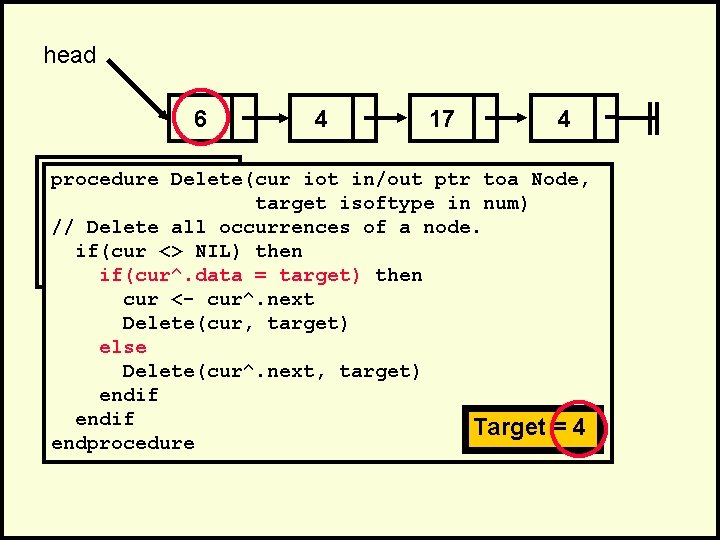head 6 4 17 4 . procedure Delete(cur iot in/out ptr toa Node, . target isoftype in num) Delete(head, // Delete all 4)occurrences of a node. . if(cur <> NIL) then. if(cur^. data = target) then cur <- cur^. next Delete(cur, target) else Delete(cur^. next, target) endif endprocedure Target = 4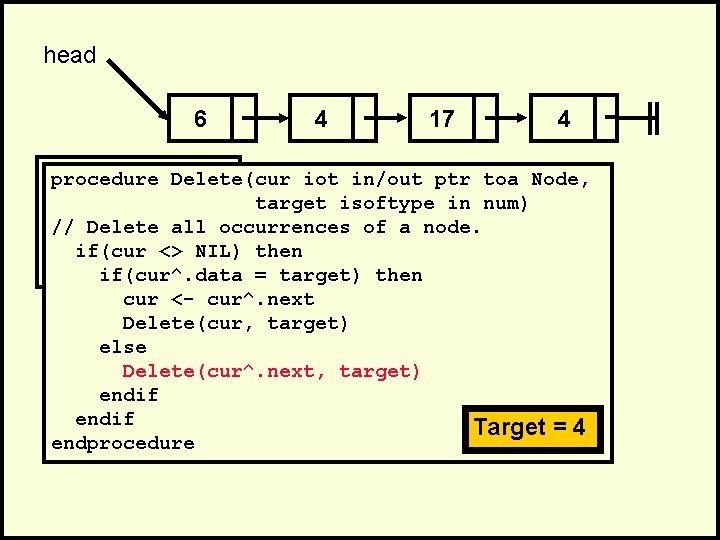head 6 4 17 4 . procedure Delete(cur iot in/out ptr toa Node, . target isoftype in num) Delete(head, // Delete all 4)occurrences of a node. . if(cur <> NIL) then. if(cur^. data = target) then cur <- cur^. next Delete(cur, target) else Delete(cur^. next, target) endif endprocedure Target = 4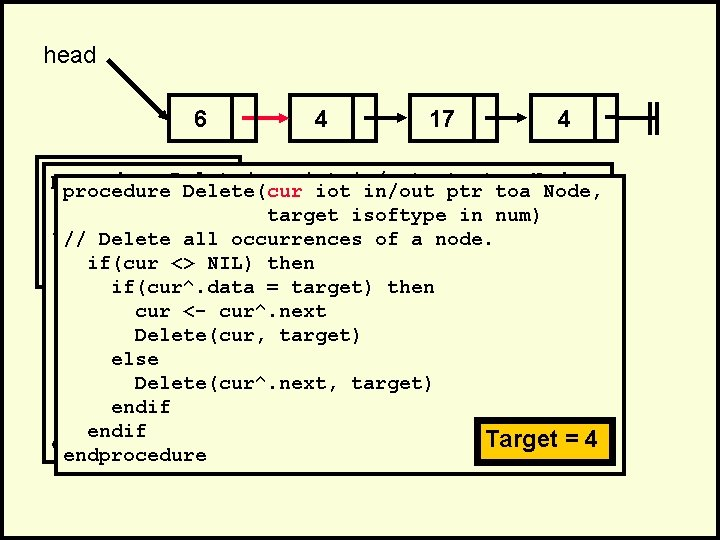head 6 4 17 4 . procedure Delete(cur iot in/out ptr toa Node, . procedure Delete(cur target isoftype in num) Delete(head, // Delete all 4)occurrences of a node. Delete all occurrences of a node. . // if(cur <> NIL) then. if(cur^. data = target) then cur <- cur^. next Delete(cur, target) else Delete(cur^. next, target) endif Target ==44 endif Target endprocedure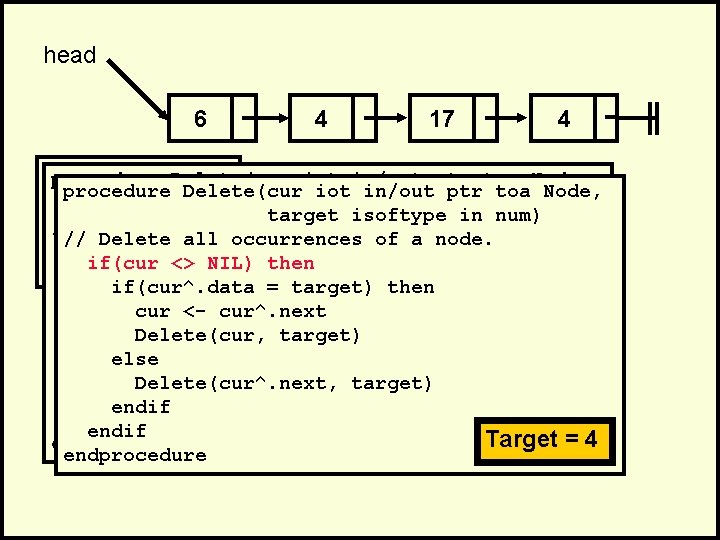head 6 4 17 4 . procedure Delete(cur iot in/out ptr toa Node, . procedure Delete(cur target isoftype in num) Delete(head, // Delete all 4)occurrences of a node. Delete all occurrences of a node. . // if(cur <> NIL) then. if(cur^. data = target) then cur <- cur^. next Delete(cur, target) else Delete(cur^. next, target) endif Target ==44 endif Target endprocedurehead 6 4 17 4 . procedure Delete(cur iot in/out ptr toa Node, . procedure Delete(cur target isoftype in num) Delete(head, // Delete all 4)occurrences of a node. Delete all occurrences of a node. . // if(cur <> NIL) then. if(cur^. data = target) then cur <- cur^. next Delete(cur, target) else Delete(cur^. next, target) endif Target ==44 endif Target endprocedure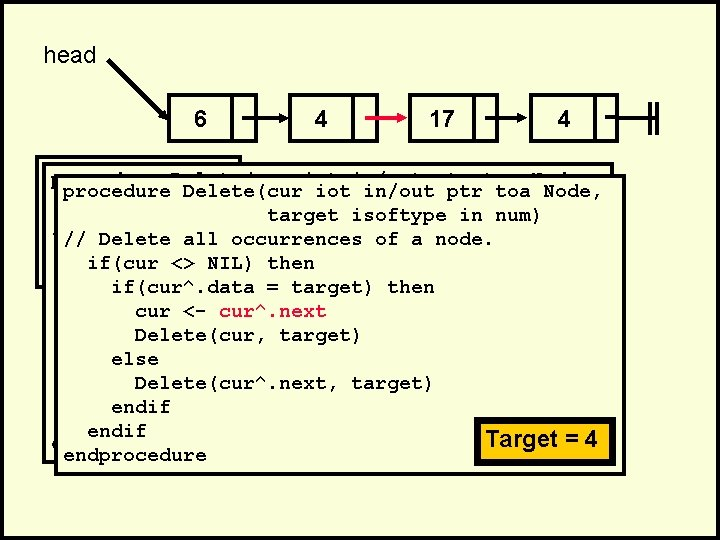head 6 4 17 4 . procedure Delete(cur iot in/out ptr toa Node, . procedure Delete(cur target isoftype in num) Delete(head, // Delete all 4)occurrences of a node. Delete all occurrences of a node. . // if(cur <> NIL) then. if(cur^. data = target) then cur <- cur^. next Delete(cur, target) else Delete(cur^. next, target) endif Target ==44 endif Target endprocedurehead 6 4 17 4 . procedure Delete(cur iot in/out ptr toa Node, . procedure Delete(cur target isoftype in num) Delete(head, // Delete all 4)occurrences of a node. Delete all occurrences of a node. . // if(cur <> NIL) then. if(cur^. data = target) then cur <- cur^. next Delete(cur, target) else Delete(cur^. next, target) endif Target ==44 endif Target endprocedurehead 6 17 4 . procedure Delete(cur iot in/out ptr toa Node, . procedure Delete(cur target isoftype in num) Delete(head, // Delete all 4)occurrences of a node. Delete all occurrences of a node. . // if(cur <> NIL) then. if(cur^. data = target) then cur <- cur^. next Delete(cur, target) else Delete(cur^. next, target) endif Target ==44 endif Target endprocedure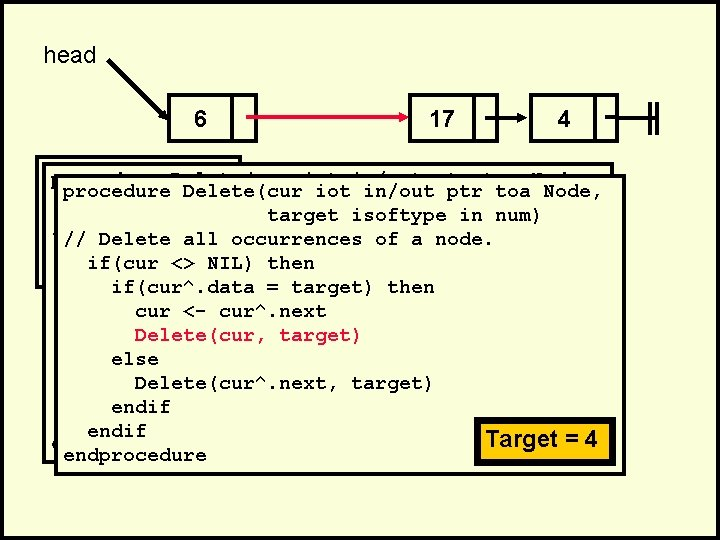head 6 17 4 . procedure Delete(cur iot in/out ptr toa Node, . procedure Delete(cur target isoftype in num) Delete(head, // Delete all 4)occurrences of a node. Delete all occurrences of a node. . // if(cur <> NIL) then. if(cur^. data = target) then cur <- cur^. next Delete(cur, target) else Delete(cur^. next, target) endif Target ==44 endif Target endprocedurehead 6 17 4 . procedure Delete(cur iot in/out ptr toa Node, . procedure Delete(cur targetiot isoftype in/outin ptr num) toa Node, target isoftype in num) Delete(head, // Delete all 4)occurrences target of isoftype a node. in num) // Delete all occurrences of a node. . // if(cur Delete <> all NIL)occurrences then of a node. if(cur <> NIL) then. if(cur^. data if(cur <> NIL) = then target) then if(cur^. data = target) then cur <- cur^. next Delete(cur, cur <- cur^. next target) Delete(cur, target) else Delete(cur^. next, target) endif endif Target ==44 endif Target endprocedure endif Target = 4 endprocedure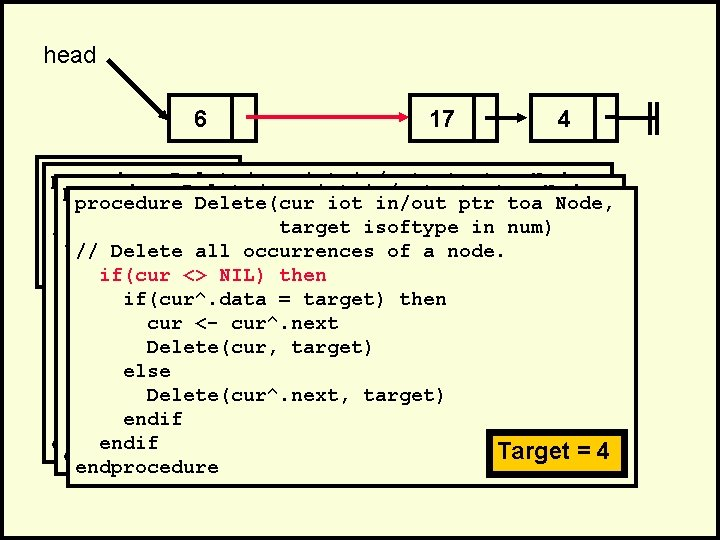head 6 17 4 . procedure Delete(cur iot in/out ptr toa Node, . procedure Delete(cur targetiot isoftype in/outin ptr num) toa Node, target isoftype in num) Delete(head, // Delete all 4)occurrences target of isoftype a node. in num) // Delete all occurrences of a node. . // if(cur Delete <> all NIL)occurrences then of a node. if(cur <> NIL) then. if(cur^. data if(cur <> NIL) = target) then if(cur^. data = target) then cur <- cur^. next Delete(cur, cur <- cur^. next target) Delete(cur, target) else Delete(cur^. next, target) endif endif Target ==44 endif Target endprocedure endif Target = 4 endprocedure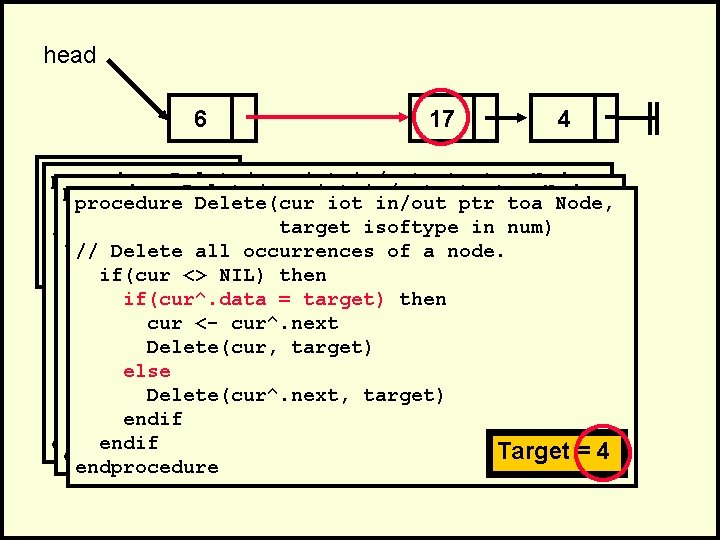head 6 17 4 . procedure Delete(cur iot in/out ptr toa Node, . procedure Delete(cur targetiot isoftype in/outin ptr num) toa Node, target isoftype in num) Delete(head, // Delete all 4)occurrences target of isoftype a node. in num) // Delete all occurrences of a node. . // if(cur Delete <> all NIL)occurrences then of a node. if(cur <> NIL) then. if(cur^. data if(cur <> NIL) = then target) then if(cur^. data = target) then cur <- cur^. next Delete(cur, cur <- cur^. next target) Delete(cur, target) else Delete(cur^. next, else target) Delete(cur^. next, target) endif Target ==44 endif Target endprocedure endif Target = 4 endprocedure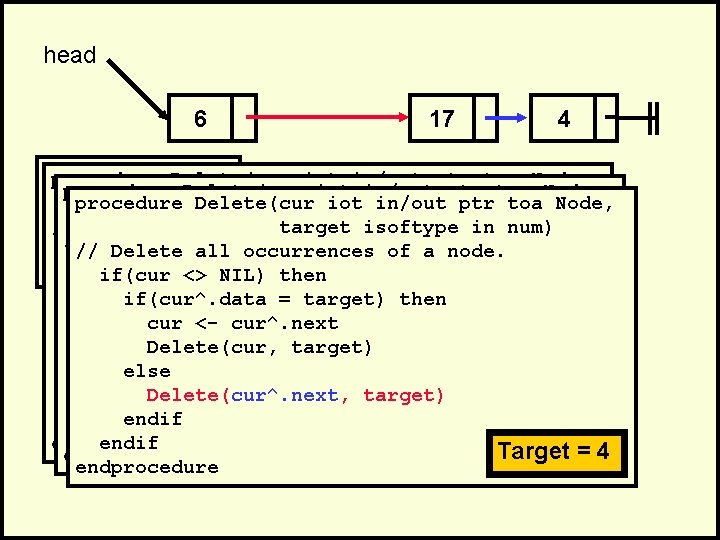head 6 17 4 . procedure Delete(cur iot in/out ptr toa Node, . procedure Delete(cur targetiot isoftype in/outin ptr num) toa Node, target isoftype in num) Delete(head, // Delete all 4)occurrences target of isoftype a node. in num) // Delete all occurrences of a node. . // if(cur Delete <> all NIL)occurrences then of a node. if(cur <> NIL) then. if(cur^. data if(cur <> NIL) = then target) then if(cur^. data = target) then cur <- cur^. next Delete(cur, cur <- cur^. next target) Delete(cur, target) else Delete(cur^. next, target) endif endif Target ==44 endif Target endprocedure endif Target = 4 endprocedure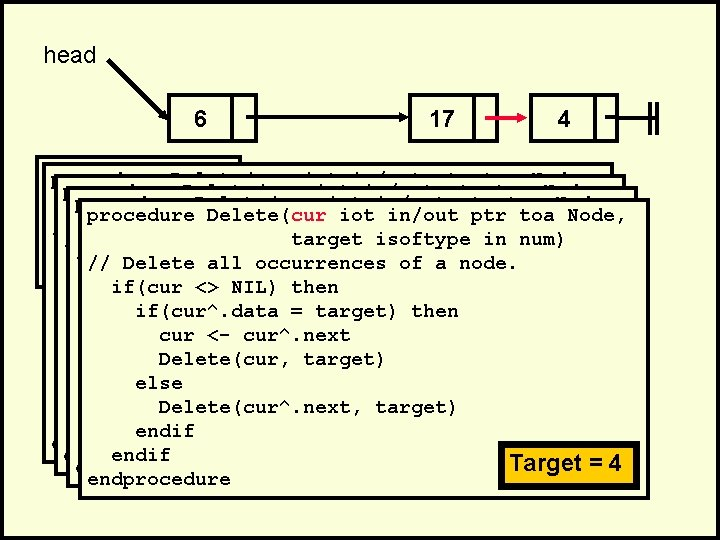head 6 17 4 . procedure Delete(cur iot in/out ptr toa Node, . procedure Delete(cur targetiot isoftype in/outin ptr num) toa Node, procedure Delete(cur target iot isoftype in/out in ptr num) toa Node, Delete(head, // Delete all 4)occurrences target of isoftype a node. in num) Delete all occurrences target of isoftype a node. in num). // if(cur // Delete <> all NIL)occurrences then of a node. . if(cur^. data if(cur <> NIL) = then target) then if(cur^. data = target) then cur <- cur^. next Delete(cur, cur <- cur^. next target) else. Delete(cur, target) Delete(cur^. next, target) else endif Delete(cur^. next, target) endif Target ==44 endif Target endprocedure endif Target ==44 endprocedure endif Target endprocedure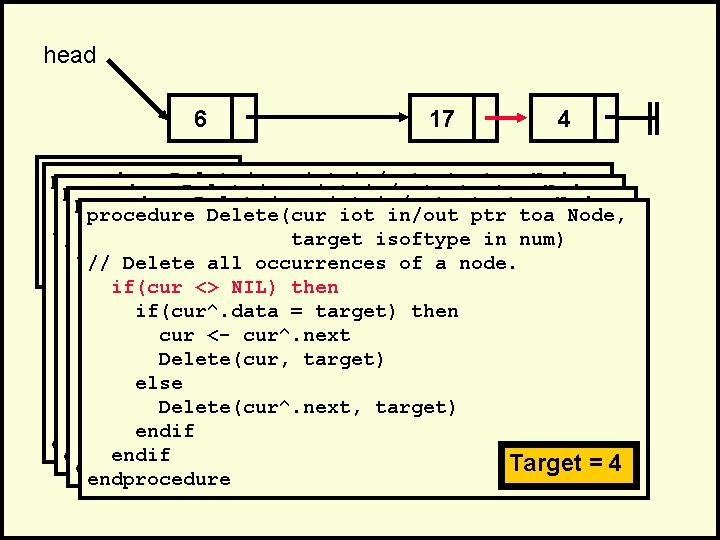head 6 17 4 . procedure Delete(cur iot in/out ptr toa Node, . procedure Delete(cur targetiot isoftype in/outin ptr num) toa Node, procedure Delete(cur target iot isoftype in/out in ptr num) toa Node, Delete(head, // Delete all 4)occurrences target of isoftype a node. in num) Delete all occurrences target of isoftype a node. in num). // if(cur // Delete <> all NIL)occurrences then of a node. . if(cur^. data if(cur <> NIL) = then target) then if(cur^. data if(cur <> NIL) = target) then if(cur^. data = target) then cur <- cur^. next Delete(cur, cur <- cur^. next target) else. Delete(cur, target) Delete(cur^. next, target) else endif Delete(cur^. next, target) endif Target ==44 endif Target endprocedure endif Target ==44 endprocedure endif Target endprocedurehead 6 17 4 . procedure Delete(cur iot in/out ptr toa Node, . procedure Delete(cur targetiot isoftype in/outin ptr num) toa Node, procedure Delete(cur target iot isoftype in/out in ptr num) toa Node, Delete(head, // Delete all 4)occurrences target of isoftype a node. in num) Delete all occurrences target of isoftype a node. in num). // if(cur // Delete <> all NIL)occurrences then of a node. . if(cur^. data if(cur <> NIL) = then target) then if(cur^. data = target) then cur <- cur^. next Delete(cur, cur <- cur^. next target) else. Delete(cur, target) Delete(cur^. next, target) else endif Delete(cur^. next, target) endif Target ==44 endif Target endprocedure endif Target ==44 endprocedure endif Target endprocedurehead 6 17 4 . procedure Delete(cur iot in/out ptr toa Node, . procedure Delete(cur targetiot isoftype in/outin ptr num) toa Node, procedure Delete(cur target iot isoftype in/out in ptr num) toa Node, Delete(head, // Delete all 4)occurrences target of isoftype a node. in num) Delete all occurrences target of isoftype a node. in num). // if(cur // Delete <> all NIL)occurrences then of a node. . if(cur^. data if(cur <> NIL) = then target) then if(cur^. data = target) then cur <- cur^. next Delete(cur, cur <- cur^. next target) else. Delete(cur, target) Delete(cur^. next, target) else endif Delete(cur^. next, target) endif Target ==44 endif Target endprocedure endif Target ==44 endprocedure endif Target endprocedure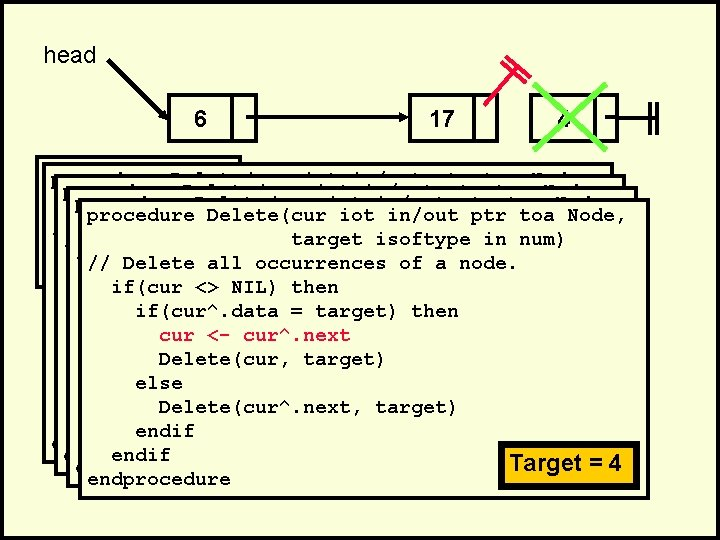head 6 17 4 . procedure Delete(cur iot in/out ptr toa Node, . procedure Delete(cur targetiot isoftype in/outin ptr num) toa Node, procedure Delete(cur target iot isoftype in/out in ptr num) toa Node, Delete(head, // Delete all 4)occurrences target of isoftype a node. in num) Delete all occurrences target of isoftype a node. in num). // if(cur // Delete <> all NIL)occurrences then of a node. . if(cur^. data if(cur <> NIL) = then target) then if(cur^. data = target) then cur <- cur^. next Delete(cur, cur <- cur^. next target) else. Delete(cur, target) Delete(cur^. next, target) else endif Delete(cur^. next, target) endif Target ==44 endif Target endprocedure endif Target ==44 endprocedure endif Target endprocedurehead 6 17 . procedure Delete(cur iot in/out ptr toa Node, . procedure Delete(cur targetiot isoftype in/outin ptr num) toa Node, procedure Delete(cur target iot isoftype in/out in ptr num) toa Node, Delete(head, // Delete all 4)occurrences target of isoftype a node. in num) Delete all occurrences target of isoftype a node. in num). // if(cur // Delete <> all NIL)occurrences then of a node. . if(cur^. data if(cur <> NIL) = then target) then if(cur^. data = target) then cur <- cur^. next Delete(cur, cur <- cur^. next target) else. Delete(cur, target) Delete(cur^. next, target) else endif Delete(cur^. next, target) endif Target ==44 endif Target endprocedure endif Target ==44 endprocedure endif Target endprocedurehead 6 17 . procedure Delete(cur iot in/out ptr toa Node, . procedure Delete(cur targetiot isoftype in/outin ptr num) toa Node, procedure Delete(cur target iot isoftype in/out in ptr num) toa Node, Delete(head, // Delete procedure all 4)Delete(cur occurrences targetiot of isoftype a in/out node. in ptr num) toa Node, // Delete all occurrences target of isoftype a node. in num). // if(cur Delete <> all NIL)occurrences thentarget of isoftype a node. in num) if(cur // Delete <> NIL) all occurrences then of a node. . if(cur^. data if(cur // Delete <> all NIL) =occurrences target) then of a node. if(cur^. data if(cur <> NIL) = then target) then if(cur^. data if(cur NIL) = then target) then cur <- <> cur^. next if(cur^. data = target) then cur <- cur^. next if(cur^. data = target) then Delete(cur, cur <- cur^. next target) else. Delete(cur, target) Delete(cur^. next, else Delete(cur, target) Delete(cur^. next, target) else endif Delete(cur^. next, target) Target = 4 endif Target ==44 endprocedure endif Target endprocedure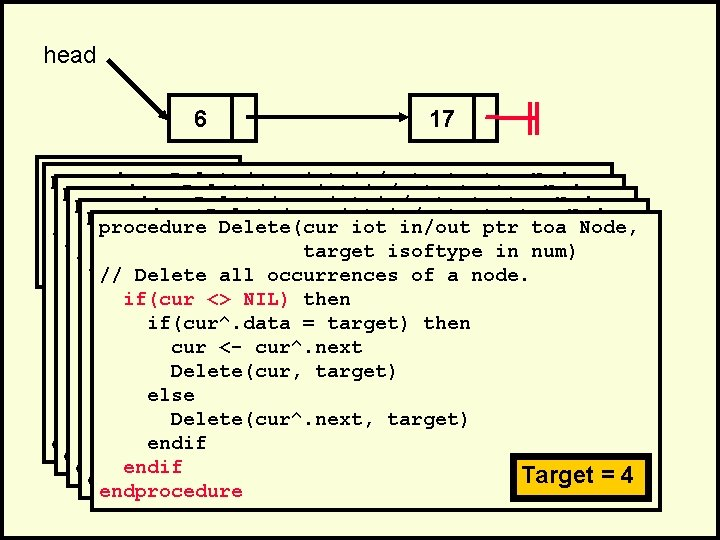head 6 17 . procedure Delete(cur iot in/out ptr toa Node, . procedure Delete(cur targetiot isoftype in/outin ptr num) toa Node, procedure Delete(cur target iot isoftype in/out in ptr num) toa Node, Delete(head, // Delete procedure all 4)Delete(cur occurrences targetiot of isoftype a in/out node. in ptr num) toa Node, // Delete all occurrences target of isoftype a node. in num). // if(cur Delete <> all NIL)occurrences thentarget of isoftype a node. in num) if(cur // Delete <> NIL) all occurrences then of a node. . if(cur^. data if(cur // Delete <> all NIL) =occurrences target) then of a node. if(cur^. data if(cur <> NIL) = then target) then if(cur^. data if(cur <> NIL) = target) then cur <- cur^. next if(cur^. data = target) then Delete(cur, cur <- cur^. next target) else. Delete(cur, target) Delete(cur^. next, else Delete(cur, target) Delete(cur^. next, target) else endif Delete(cur^. next, target) Target = 4 endif Target ==44 endprocedure endif Target endprocedure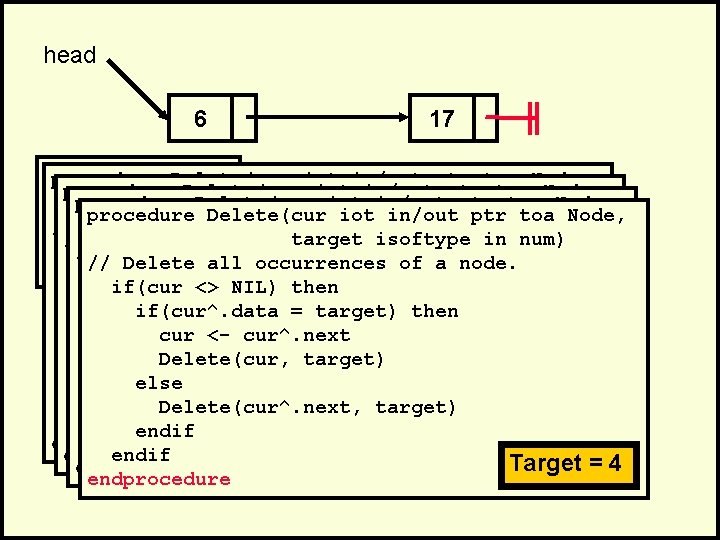head 6 17 . procedure Delete(cur iot in/out ptr toa Node, . procedure Delete(cur targetiot isoftype in/outin ptr num) toa Node, procedure Delete(cur target iot isoftype in/out in ptr num) toa Node, Delete(head, // Delete all 4)occurrences target of isoftype a node. in num) Delete all occurrences target of isoftype a node. in num). // if(cur // Delete <> all NIL)occurrences then of a node. . if(cur^. data if(cur <> NIL) = then target) then if(cur^. data = target) then cur <- cur^. next Delete(cur, cur <- cur^. next target) else. Delete(cur, target) Delete(cur^. next, target) else endif Delete(cur^. next, target) endif Target ==44 endif Target endprocedure endif Target ==44 endprocedure endif Target endprocedure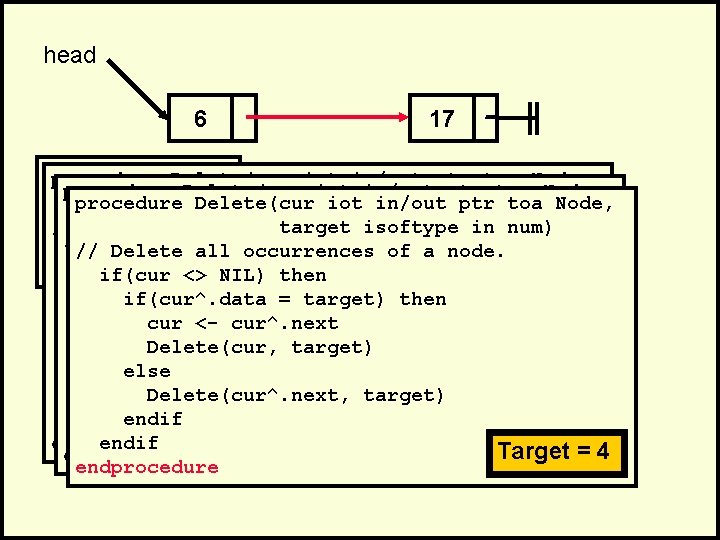head 6 17 . procedure Delete(cur iot in/out ptr toa Node, . procedure Delete(cur targetiot isoftype in/outin ptr num) toa Node, target isoftype in num) Delete(head, // Delete all 4)occurrences target of isoftype a node. in num) // Delete all occurrences of a node. . // if(cur Delete <> all NIL)occurrences then of a node. if(cur <> NIL) then. if(cur^. data if(cur <> NIL) = then target) then if(cur^. data = target) then cur <- cur^. next Delete(cur, cur <- cur^. next target) Delete(cur, target) else Delete(cur^. next, target) endif endif Target ==44 endif Target endprocedure endif Target = 4 endprocedurehead 6 17 . procedure Delete(cur iot in/out ptr toa Node, . procedure Delete(cur target isoftype in num) Delete(head, // Delete all 4)occurrences of a node. Delete all occurrences of a node. . // if(cur <> NIL) then. if(cur^. data = target) then cur <- cur^. next Delete(cur, target) else Delete(cur^. next, target) endif Target ==44 endif Target endprocedurehead 6 17 . procedure Delete(cur iot in/out ptr toa Node, . target isoftype in num) Delete(head, // Delete all 4)occurrences of a node. . if(cur <> NIL) then. if(cur^. data = target) then cur <- cur^. next Delete(cur, target) else Delete(cur^. next, target) endif endprocedure Target = 4